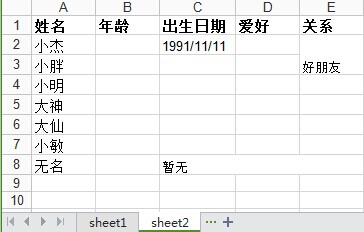# python中使用xlrd、xlwt操作excel表格详解

更新时间：2015年01月29日 11:05:47   投稿：junjie

python操作excel主要用到xlrd和xlwt这两个库，即xlrd是读excel，xlwt是写excel的库。可从这里下载https://pypi.python.org/pypi。下面分别记录python读和写excel.

python读excel――xlrdpython 对 excel基本的操作如下：

```# -*- coding: utf-8 -*-
import xlrd
import xlwt
from datetime import date,datetime

def read_excel():
# 打开文件
workbook = xlrd.open_workbook(r'F:\demo.xlsx')
# 获取所有sheet
print workbook.sheet_names() # [u'sheet1', u'sheet2']
sheet2_name = workbook.sheet_names()

# 根据sheet索引或者名称获取sheet内容
sheet2 = workbook.sheet_by_index(1) # sheet索引从0开始
sheet2 = workbook.sheet_by_name('sheet2')

# sheet的名称，行数，列数
print sheet2.name,sheet2.nrows,sheet2.ncols

# 获取整行和整列的值（数组）
rows = sheet2.row_values(3) # 获取第四行内容
cols = sheet2.col_values(2) # 获取第三列内容
print rows
print cols

# 获取单元格内容
print sheet2.cell(1,0).value.encode('utf-8')
print sheet2.cell_value(1,0).encode('utf-8')
print sheet2.row(1).value.encode('utf-8')

# 获取单元格内容的数据类型
print sheet2.cell(1,0).ctype

if __name__ == '__main__':
read_excel()

```1、python读取excel中单元格内容为日期的方式

python读取excel中单元格的内容返回的有5种类型，即上面例子中的ctype:

```ctype : 0 empty,1 string, 2 number, 3 date, 4 boolean, 5 error
```

```>>> sheet2.cell(2,2).ctype #1990/2/22
>>> sheet2.cell(2,1).ctype #24
>>> sheet2.cell(2,0).ctype #小胖
>>> sheet2.cell(2,4).ctype #空值（这里是合并单元格的原因）
>>> sheet2.cell(2,2).value #1990/2/22
33656.0
>>> xlrd.xldate_as_tuple(sheet2.cell_value(2,2),workbook.datemode)
(1992, 2, 22, 0, 0, 0)
>>> date_value = xlrd.xldate_as_tuple(sheet2.cell_value(2,2),workbook.datemode)
>>> date_value
(1992, 2, 22, 0, 0, 0)
>>> date(*date_value[:3])
datetime.date(1992, 2, 22)
>>> date(*date_value[:3]).strftime('%Y/%m/%d')
'1992/02/22'

```

```if (sheet.cell(row,col).ctype == 3):
date_value = xlrd.xldate_as_tuple(sheet.cell_value(rows,3),book.datemode)
date_tmp = date(*date_value[:3]).strftime('%Y/%m/%d')

```

```>>> sheet2.col_values(4)
[u'\u5173\u7cfb', u'\u597d\u670b\u53cb', '', u'\u540c\u5b66', '', '', u'\u4e00\u4e2a\u4eba', '']
>>> for i in range(sheet2.nrows):
print sheet2.col_values(4)[i]

>>> sheet2.row_values(7)
[u'\u65e0\u540d', 20.0, u'\u6682\u65e0', '', '']
>>> for i in range(sheet2.ncols):
print sheet2.row_values(7)[i]

20.0

>>>

```

2、读取合并单元格的内容

```>>> print sheet2.col_values(4)

>>> print sheet2.row_values(7)

>>> sheet2.merged_cells # 明明有合并的单元格，为何这里是空
[]

```

3、获取合并的单元格

```>>> workbook = xlrd.open_workbook(r'F:\demo.xlsx',formatting_info=True)
>>> sheet2 = workbook.sheet_by_name('sheet2')
>>> sheet2.merged_cells
[(7, 8, 2, 5), (1, 3, 4, 5), (3, 6, 4, 5)]

```

merged_cells返回的这四个参数的含义是：(row,row_range,col,col_range),其中[row,row_range)包括row,不包括row_range,col也是一样，即(1, 3, 4, 5)的含义是：第1到2行（不包括3）合并，(7, 8, 2, 5)的含义是：第2到4列合并。

```>>> print sheet2.cell_value(1,4)  #(1, 3, 4, 5)

>>> print sheet2.cell_value(3,4)  #(3, 6, 4, 5)

>>> print sheet2.cell_value(7,2)  #(7, 8, 2, 5)

```

```>>> merge = []
>>> for (rlow,rhigh,clow,chigh) in sheet2.merged_cells:
merge.append([rlow,clow])

>>> merge
[[7, 2], [1, 4], [3, 4]]
>>> for index in merge:
print sheet2.cell_value(index,index)

>>>
```

python写excel――xlwt```'''

'''

def set_style(name,height,bold=False):
style = xlwt.XFStyle() # 初始化样式

font = xlwt.Font() # 为样式创建字体
font.name = name # 'Times New Roman'
font.bold = bold
font.color_index = 4
font.height = height

# borders= xlwt.Borders()
# borders.left= 6
# borders.right= 6
# borders.top= 6
# borders.bottom= 6

style.font = font
# style.borders = borders

return style

#写excel
def write_excel():
f = xlwt.Workbook() #创建工作簿

'''
创建第一个sheet:
sheet1
'''
sheet1 = f.add_sheet(u'sheet1',cell_overwrite_ok=True) #创建sheet
row0 = [u'业务',u'状态',u'北京',u'上海',u'广州',u'深圳',u'状态小计',u'合计']
column0 = [u'机票',u'船票',u'火车票',u'汽车票',u'其它']
status = [u'预订',u'出票',u'退票',u'业务小计']

#生成第一行
for i in range(0,len(row0)):
sheet1.write(0,i,row0[i],set_style('Times New Roman',220,True))

#生成第一列和最后一列(合并4行)
i, j = 1, 0
while i < 4*len(column0) and j < len(column0):
sheet1.write_merge(i,i+3,0,0,column0[j],set_style('Arial',220,True)) #第一列
sheet1.write_merge(i,i+3,7,7) #最后一列"合计"
i += 4
j += 1

sheet1.write_merge(21,21,0,1,u'合计',set_style('Times New Roman',220,True))

#生成第二列
i = 0
while i < 4*len(column0):
for j in range(0,len(status)):
sheet1.write(j+i+1,1,status[j])
i += 4

f.save('demo1.xlsx') #保存文件

if __name__ == '__main__':
#generate_workbook()
#read_excel()
write_excel()

```

write_merge(x, x + m, y, w + n, string, sytle)
x表示行，y表示列，m表示跨行个数，n表示跨列个数，string表示要写入的单元格内容，style表示单元格样式。其中，x，y，w，h，都是以0开始计算的。```'''
创建第二个sheet:
sheet2
'''
sheet2 = f.add_sheet(u'sheet2',cell_overwrite_ok=True) #创建sheet2
row0 = [u'姓名',u'年龄',u'出生日期',u'爱好',u'关系']
column0 = [u'小杰',u'小胖',u'小明',u'大神',u'大仙',u'小敏',u'无名']

#生成第一行
for i in range(0,len(row0)):
sheet2.write(0,i,row0[i],set_style('Times New Roman',220,True))

#生成第一列
for i in range(0,len(column0)):
sheet2.write(i+1,0,column0[i],set_style('Times New Roman',220))

sheet2.write(1,2,'1991/11/11')
sheet2.write_merge(7,7,2,4,u'暂无') #合并列单元格
sheet2.write_merge(1,2,4,4,u'好朋友') #合并行单元格

f.save('demo1.xlsx') #保存文件
```